You are currently offline. Some features of the site may not work correctly.

# Factor-critical graph

Known as: Blossom (graph theory)
In graph theory, a mathematical discipline, a factor-critical graph (or hypomatchable graph) is a graph with n vertices in which every subgraph of n… Expand
Wikipedia

## Papers overview

Semantic Scholar uses AI to extract papers important to this topic.
2015
2015
• Discret. Math.
• 2015
• Corpus ID: 40800768
We show that a factor-critical graph of order n has exactly n near-perfect matchings if and only if it is a connected graph whose… Expand
Is this relevant?
2011
2011
• Graphs Comb.
• 2011
• Corpus ID: 19317969
A graph is total domination edge-critical if the addition of any edge decreases the total domination number, while a graph with… Expand
Is this relevant?
2010
2010
• Discrete Math., Alg. and Appl.
• 2010
• Corpus ID: 39382728
A vertex subset S of a graph G = (V, E) is a double dominating set for G if |N[v]∩S| ≥ 2 for each vertex v ∈ V, where N[v] = {u… Expand
Is this relevant?
2007
2007
• Discret. Math.
• 2007
• Corpus ID: 27576255
A graph G is said to be [email protected] if the size of any minimum dominating set of vertices is k, but if any edge is added to… Expand
•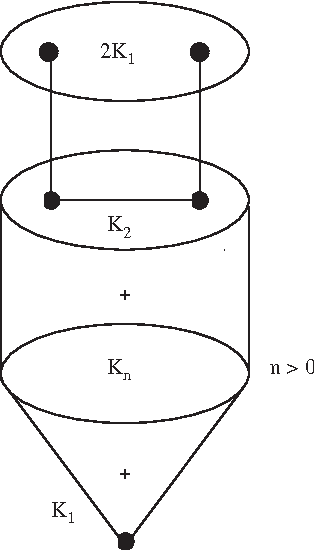•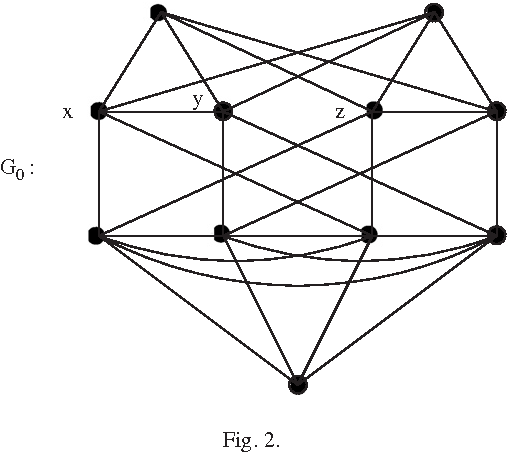•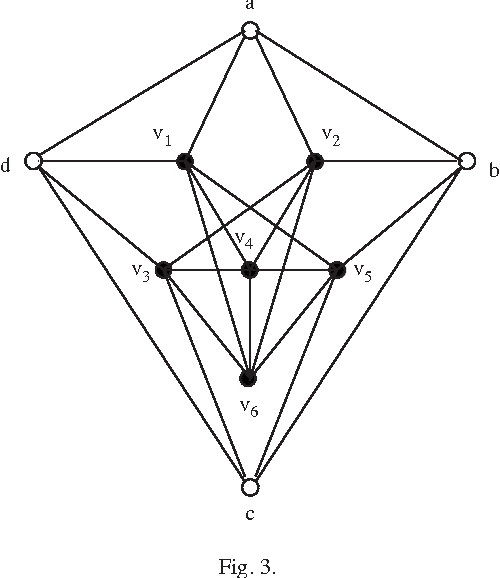•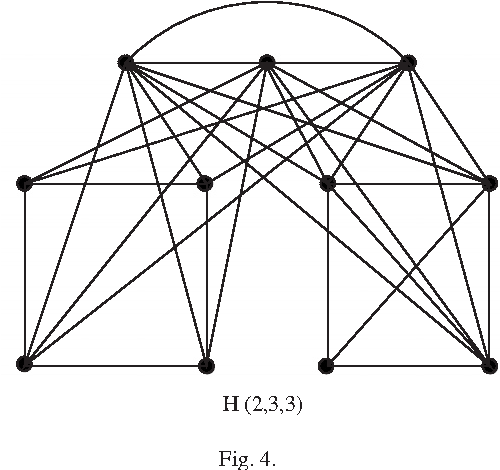•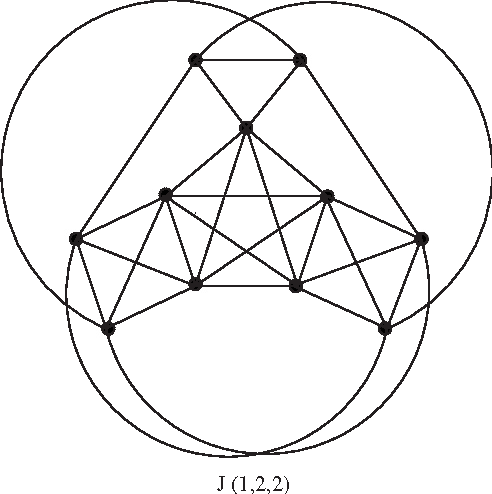Is this relevant?
2004
2004
• Australas. J Comb.
• 2004
• Corpus ID: 9247485
In this paper, we show a necessary and sufficient condition which characterizes all factor critical graphs. Using this necessary… Expand
Is this relevant?
2004
2004
• Discret. Math.
• 2004
• Corpus ID: 21852097
A graph G is said to be [email protected] if the size of any minimum dominating set of vertices is k, but if any edge is added to… Expand
•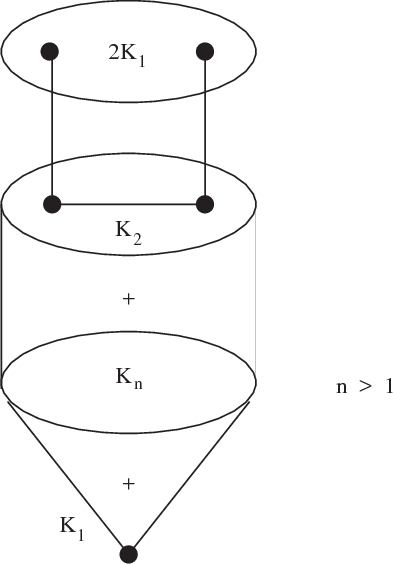•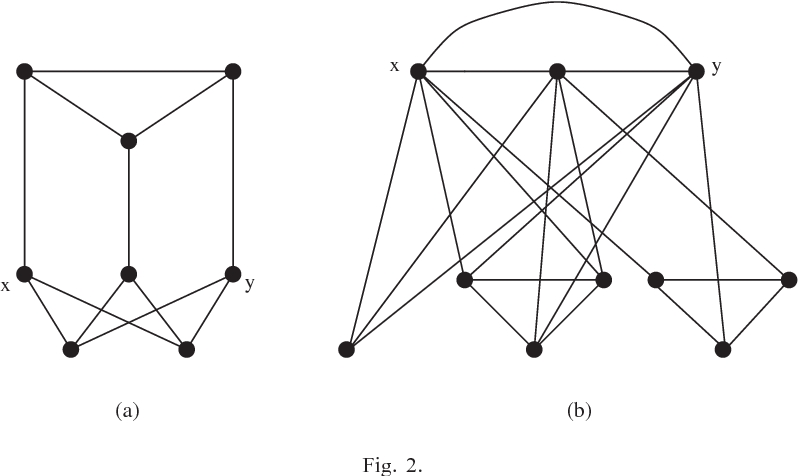•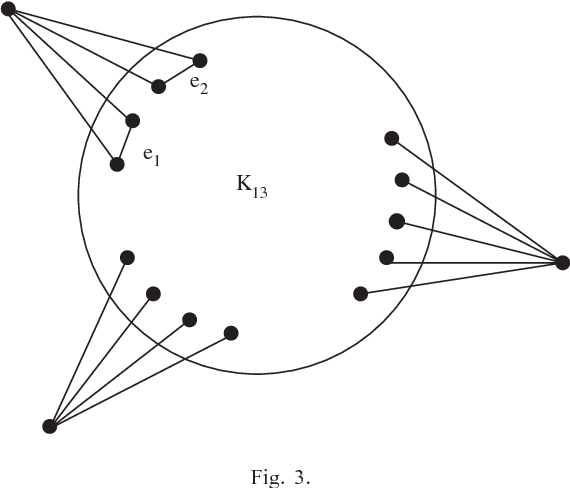•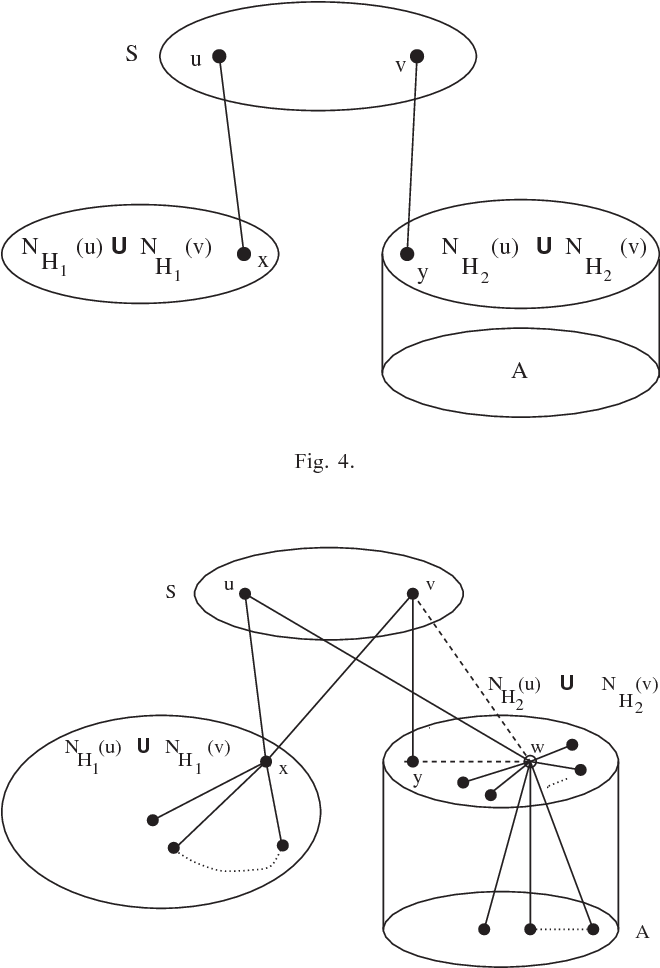•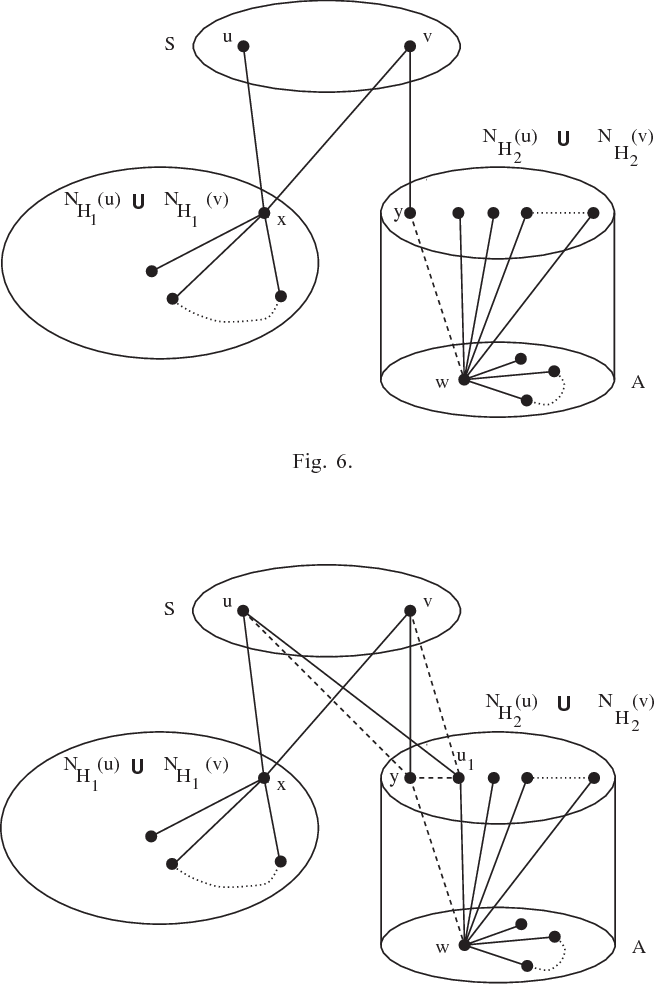Is this relevant?
2002
2002
• Discret. Math.
• 2002
• Corpus ID: 34451404
Abstract A matching of a graph is a near-perfect matching if it covers all but one vertex. A connected graph G is said to be… Expand
Is this relevant?
1998
1998
• Australas. J Comb.
• 1998
• Corpus ID: 44414741
A graph G of order n is k-factor-critical, where k is an integer of the same parity as n with 0 ::; k ::; n, if G X has a perfect… Expand
Is this relevant?
1992
1992
• Discret. Math.
• 1992
• Corpus ID: 6641485
A total cover of a graph G is a subset of V(G)∪E(G) which covers all elements of V(G)∪E(G). The total covering number α2(G) of a… Expand
Is this relevant?
1990
1990
A subsetJ of edges of a connected undirected graphG=(V, E) is called ajoin if |C∩J|≤|C|/2 for every circuitC ofG. Answering a… Expand
Is this relevant?